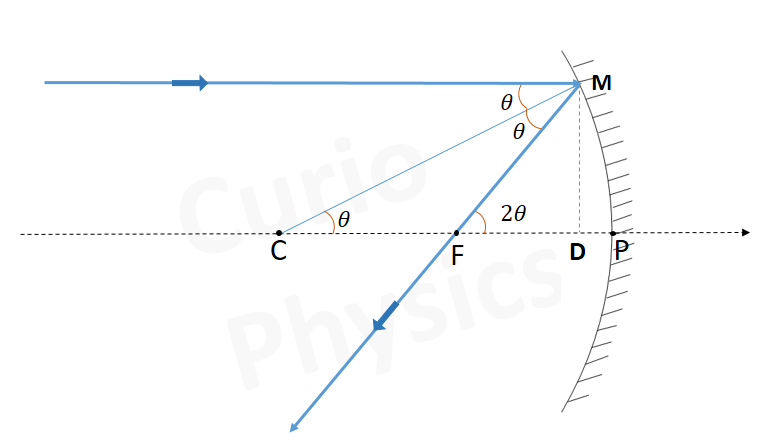# Relation between focal length and radius of curvature

## (f = R/2)

Relation between focal length and radius of curvature :- Let C be the center of curvature of the mirror. Consider a ray parallel to the principal axis striking the mirror at M. Then CM will be perpendicular to the mirror at M. Let θ be the angle of incidence, and MD be the perpendicular from M on the principal axis. Then,

For Concave MirrorFor Convex Mirror∠MCP = θ and ∠MFP = 2θ

tanθ = MD/CD and tan 2θ = MD/FD

For small θ, which is true for paraxial rays, tanθ ≈ θ and tan 2θ ≈ 2θ.

Therefore,

MD/FD = 2(MD/CD)

Or FD = CD/2

Now, for small values of θ, the point D is very close to the point P. Therefore, FD = f and CD = R. Then

f = R/2

Next Topic :- Mirror Formula.